Some knowledge and practice will add up quickly as you learn the ins and outs of addition.Author
Taylor HartleyExpert Reviewer

Published: September 1, 2023Some knowledge and practice will add up quickly as you learn the ins and outs of addition.Author
Taylor HartleyExpert Reviewer

Published: September 1, 2023Some knowledge and practice will add up quickly as you learn the ins and outs of addition.Author
Taylor HartleyExpert Reviewer

Published: September 1, 2023Key takeaways

• Addition is at the heart of most math – To understand pretty much any other aspect of math, you need to wrap your head around addition first.
• Plenty of strategies can help you along the way – Whether you use visual aids, basic numerals, or even your fingers, tackle addition problems in many different ways.
• Practice adds up – Once you understand the basic concepts of addition, you’ll be adding up bigger and bigger numbers in no time.

Aside from simple counting, addition is the most basic and fundamental mathematical skill you can learn. Before you can subtract, multiply, and divide, you need to be able to combine numbers together through the power of addition. It’s actually a skill most people use daily, whether they are paying bills, cooking a meal, scheduling different activities, or just figuring out how much candy they’ve eaten in a day.

No matter how much math you plan on doing in your life, make no mistake – everybody needs to know addition! Let’s take a deeper dive into this valuable skill.Addition is all about finding the sum, the combined value of two or more numbers. We call numbers we are adding together “addends”, but most people hardly ever use that term.

To find the sum of some addends, you take a number like 5 and then another number, like 7, and try to figure out what number you get if you combine those two. In this case, 5 + 7 will get you 12.

The + symbol is called the “plus sign,” and it is the symbol we use to show that we need to add two numbers together. We use an equal sign, which looks like =, to show what the value of the added numbers is. Get used to these symbols – you’re going to be seeing them a lot!

## How to add two numbers togetherThere are a few options to help you find the sum of numbers. Depending on how your mind works, some of these options will make more sense than others. Just feel free to try them all out and see what works best for you.

Let’s get started looking at how to find the sum of two numbers. We’ll keep things simple for now.

### Addition using a number lineA number line is a simple visual representation of numbers counting from 1 to whatever value you’d like. An easy way to figure out a sum is to use a number line.

Start by looking at your addition problem. Let’s look back at the example from earlier, 5 + 7. Look at the first number in the problem, 5, and count that many spaces on the number line. You’re halfway done already!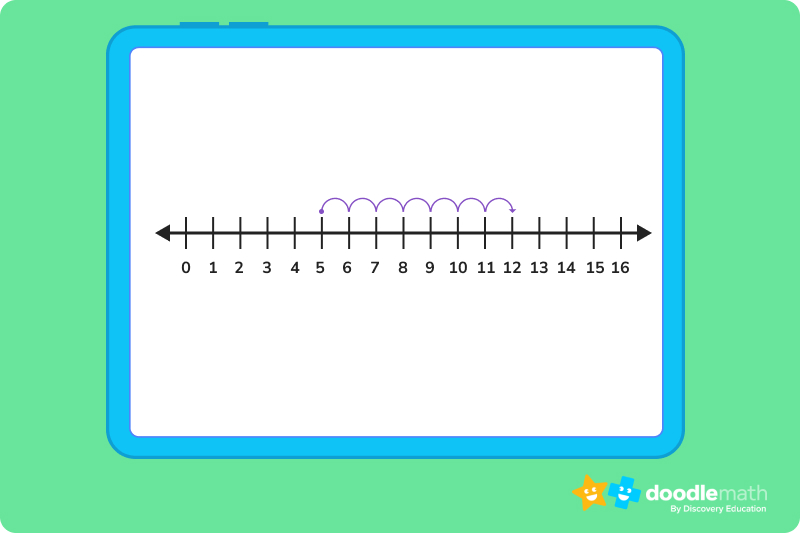Now, look at the second number in the problem, which is 7. Keeping your place on the number line that you found with the first number, count out 7 more spaces on the line. 5, 6, 7… all the way to 12!

Guess what? You just found the sum of 5 and 7!If you are trying to find the sum of smaller numbers and you don’t have any paper handy for a number line, you can just use your fingers. Let’s do this with a new addition problem, 3 + 6. Start with your hands balled up into fists. As you count out the numbers, you will hold up fingers to help you keep track, just like with spaces on a number line.

Hold out your left hand. We’re going to start with the first number again, which is 3. Count it out — 1, 2, 3 fingers. Great, now let’s handle that second number, 6. On the left hand, keep those 3 fingers up but start counting to 6 using the fingers you haven’t raised yet.

1… 2…

Now all the fingers on your left hand should be up. But wait, you are all out of fingers! Well, you can move onto your right hand. Keep counting. You can get all the way to 6 by using the 2 fingers you had on your left hand and 4 on your right. Now, count how many fingers you are holding up. You should see 9 fingers total, which is the sum of 3 and 6!

For sums greater than 10, you will need to “carry” the one mentally and start over by lowering your fingers. We’ll talk more about “carrying” in the next section.Eventually, you will need to start adding up larger and larger numbers. That means eventually the number line will get a little too long, and you will quickly run out of fingers (and toes!) for counting. You will need to rely on regrouping or carrying numbers to handle larger addition problems.

Let’s say you need to find the sum of 24 and 32. Things are about to get a little tricky, so let’s try to arrange these numbers to make things easier to understand. We will stack them up on top of each other so we can handle one part of each number at a time. It will look like this: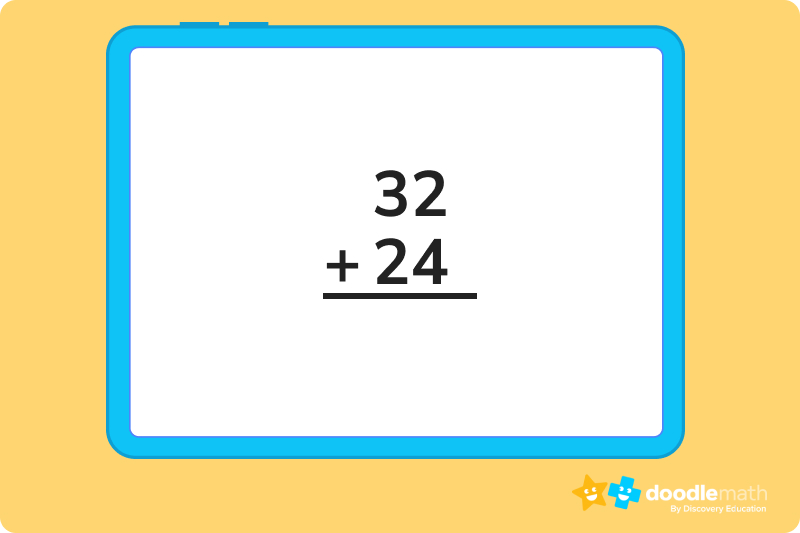Notice how the larger number is on top. It doesn’t matter which number goes on top in addition, but putting the larger number up there is a good habit to get into because of how things like subtraction work. We’ll worry about that later, though.

Now that we have the numbers lined up, we are going to add one column at a time, starting with the ones place. Let’s start by adding 2 and 4 together. For this you can try using the number line or your fingers again. 2 + 4 = 6, so let’s write a 6 down below the 2 and the 4. Like this: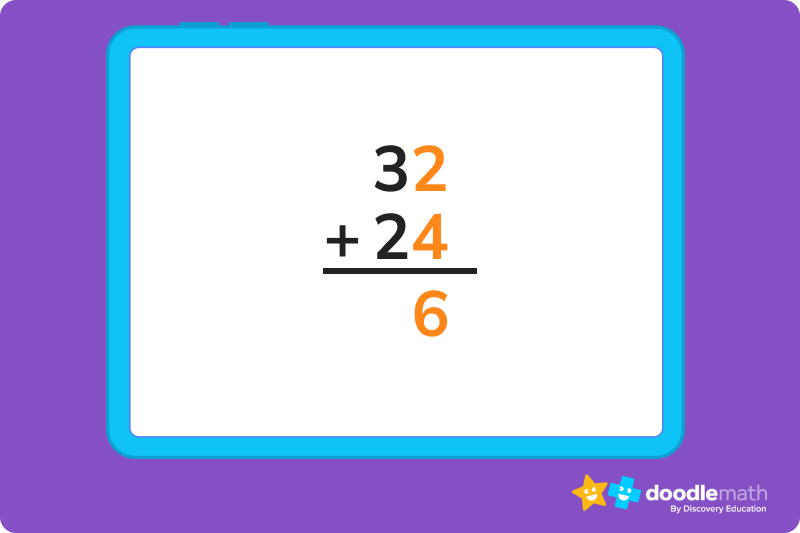Drawing a line between the numbers you are adding and the sum you are working on will help you keep the numbers straight.

Now that we have added the numbers in the ones place, let’s move onto the tens. Add up 3 and 2 with your number line or fingers and write it below in the tens space next to the 6. 3 + 2 = 5, so let’s drop that down below.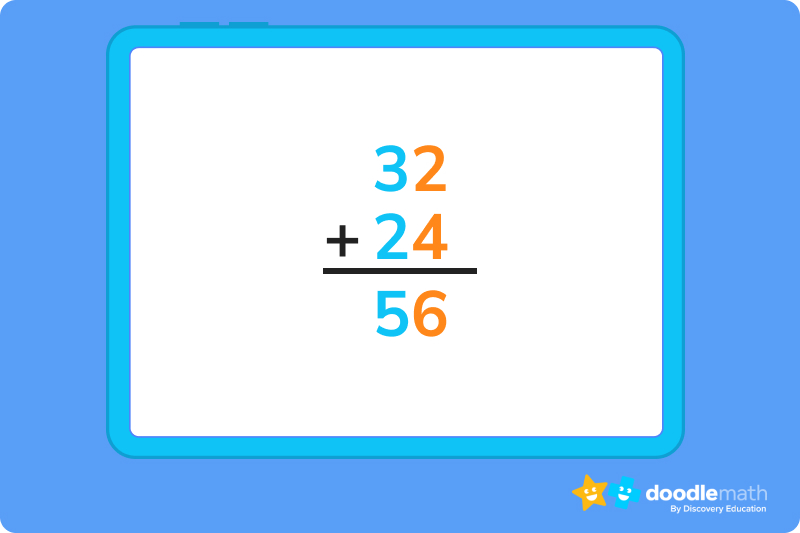And there you have it! 32 plus 24 equals 56.

It does get a little more complicated sometimes, though.

Take a look at this problem next. What is 27 + 15?

Let’s set that up just like before.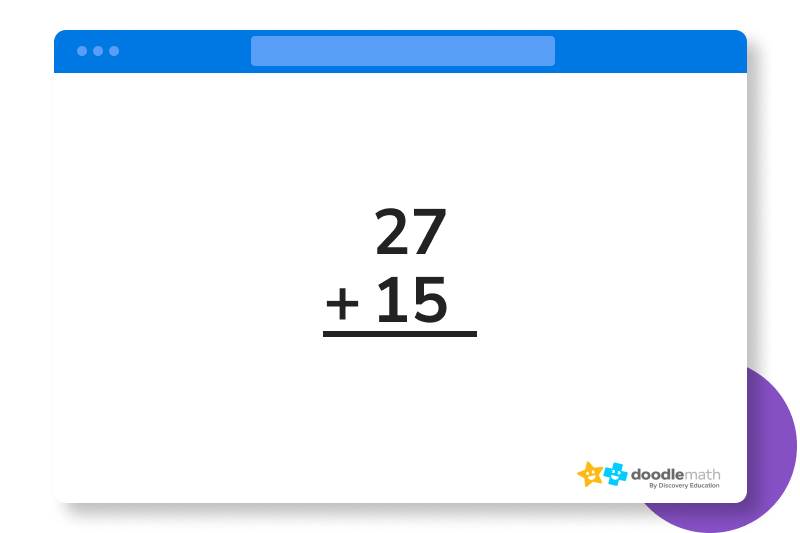Just like before, we will start with the ones place. 7 + 5. That will get you to 12, but here’s the problem. You can’t just write 12 down below like you did with the 6 from the last problem because that would take up the spot where you would add the 2 and the 1 in the tens place, right?

What you have to do is “carry” the 1 in the 12 you got from adding 7 and 5. That “1” in the number is basically just 10. You can think of 12 as being 10 + 2. That means you can put the 2 below just like before, and move the 1 to above the 27.

It’s easier to just see it in action: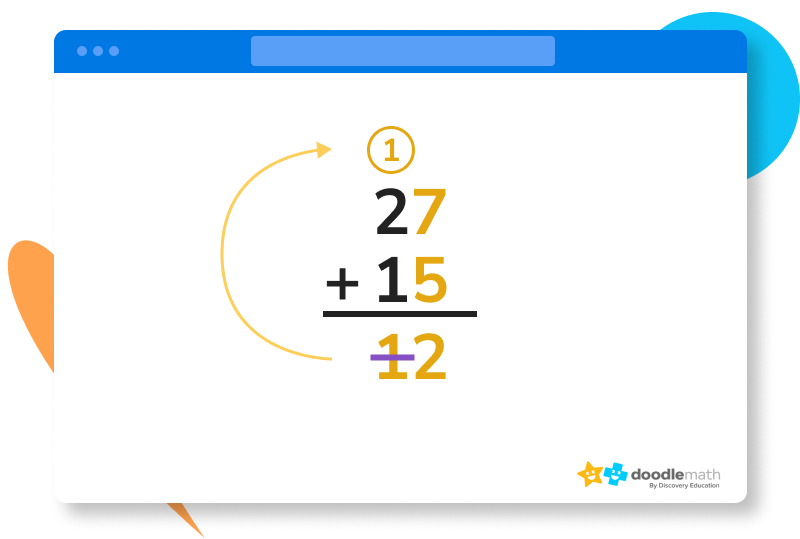Remember, that 1 up there is basically just a 10, so you are adding 3 numbers now, but it’s nothing you can’t handle. Let’s add up those numbers in the tens spot now. 1 + 2 + 1. 1 + 2 will get you 3, and then you add 1 to that to get 4.

Write that down below.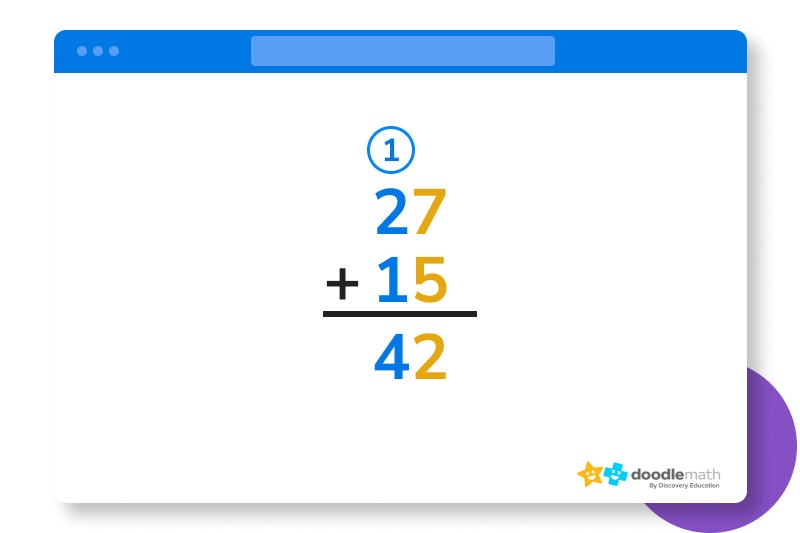And there you have it! 27 plus 15 equals 42. You can use the “carrying” technique as many times as you need to in an addition problem. Any time a column adds up to more than 9, you will carry the 1 in the tens place and place it over the next column.

Here’s an example of this process going into the hundreds place.Add up the numbers in the ones first. 7 + 5 = 12, so the 1 is carried.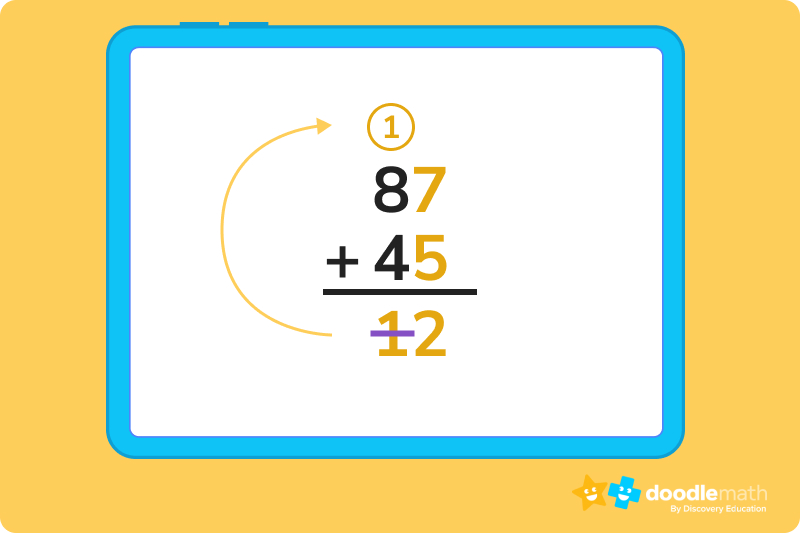Time to add up the numbers in the tens place.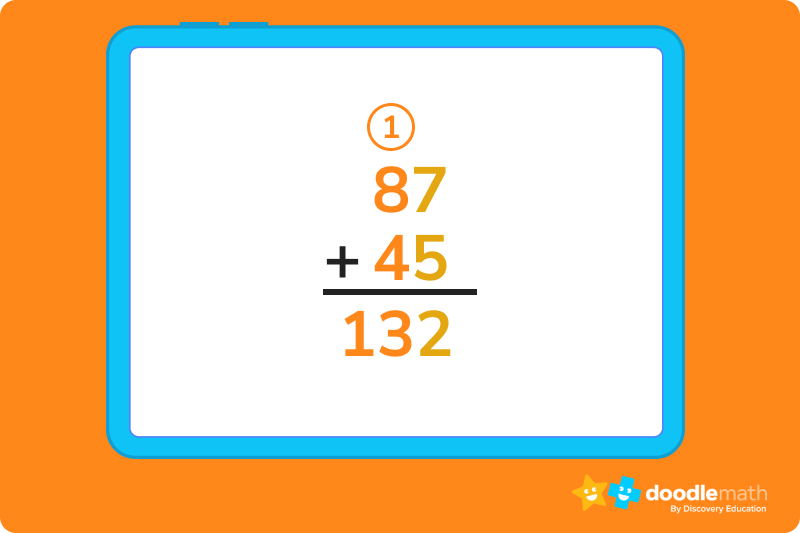Note that if you were adding up numbers that had hundreds, you would need to carry the 1 again until you run out of columns.As you might expect, addition can get even more complex since there are so many different things you can add. Fortunately, you won’t have to worry about more complicated addition until later in school, but it is good to go ahead and start thinking about these ideas.

Especially with things like cooking and baking, with 1/2 a teaspoon here and 1/2 a tablespoon there, you’ll need to figure out how to add fractions together or your cake might turn into a muffin.

To find the sum of two fractions, there are a few steps you have to follow:

• Check to see if the fractions are “like” fractions. Like fractions have the same denominators (the numbers on the bottom of the fraction). If they are, you can skip to bullet number 4. If they’re not like fractions, move on to the second bullet.
• Find the lowest common denominator. When the fractions are unlike, we need to find the lowest common denominator, or LCD. We’ll learn how to do that below.
• Make the two fractions “like” fractions. Convert each fraction into an equivalent fraction, or like fraction, having the denominator equal to the LCD found in the last step.
• Add the numbers on top. After both fractions are like fractions, add their numerators, or the number on top.
• Make it simple. Finally, reduce the fraction to the simplest form if needed.

Let’s see this in action! If a recipe calls for 1/2 cup sugar and 2/3 cup milk, how many cups do you have in total?

First, we know that the fraction for sugar = 1/2

Second, we know that the fraction for milk = 2/3

So we are trying to find out 1/2 + 2/3 = ?

The LCD of 3 and 2 is 6. How do we know this? Well, for this part, you need to know some multiplication, which is a skill you will learn more about later, but just in case you are a little ahead, here’s what that looks like.

To find the LCD, multiply the two denominators together. You will also need to multiply the numerators (the numbers on top) by the denominators.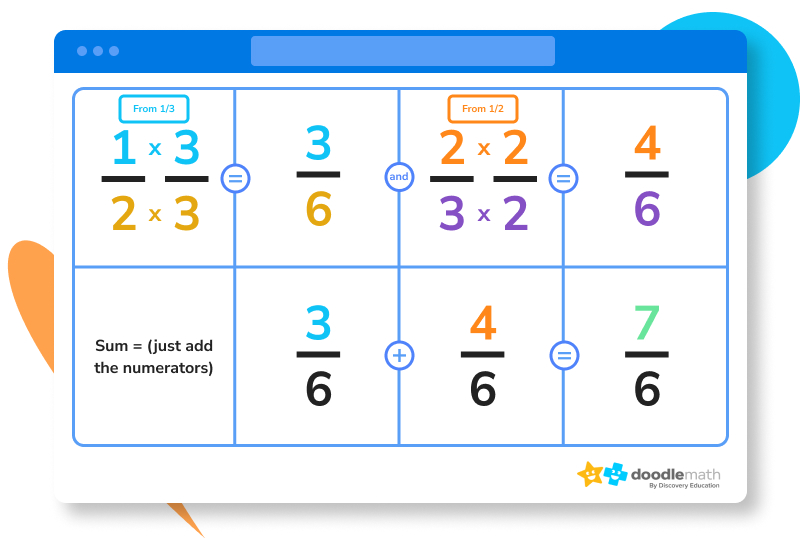There you have it! 1/2 + 2/3 = 7/6

To find the sum of two decimal numbers, there are a few steps that you have to follow:

• Convert unlike decimals into like decimals. This is easier than making fractions “like”.
• Arrange the numbers carefully. Place the decimal numbers so that the tens digit is below the other tens digit, the ones below the ones, the decimal point below the decimal point, and so on. This is just an extension of how we arranged the numbers when learning about carrying the 1 earlier.
• Add the numbers up. Use your carrying skills when you have to.
• Make sure you put the decimal in the right place. Place the decimal point in the sum, or the answer, right below the decimal point in the original decimal numbers.

Time to see this in action, too!

Let’s find the sum of 2.36 and 6.721.

We will first need to convert both the decimal numbers into like decimals by adding zeros to fill in the missing places so that each number has the same number of digits behind the decimal. 6.721 has 3 digits behind the decimal, but 2.36 only has 2. Just add some zeros to make them even, which will make 2.36 into 2.360. This doesn’t change how much the number is, but it will make adding much easier.

Next, add them like whole numbers, moving from right to left, carrying the 1 if and when needed. Don’t forget to put the decimal in your answer!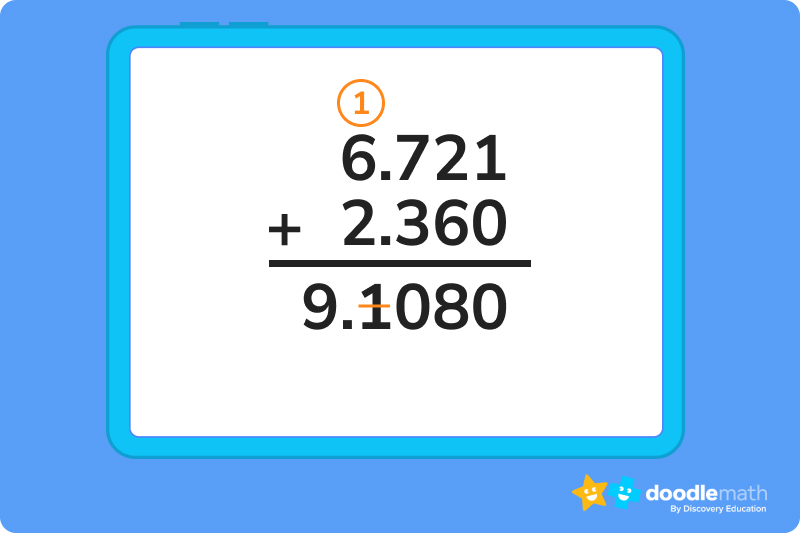The total is 9.081!

Ready to give it a go?

Now that we know how to find the sum of multiple numbers using different methods, we’re ready to put these new skills to the test! Work through the following problems on your own. Feel free to look back at the practice problems above if you get stuck, or if you need a quick reminder on how to find the sum.

You may find yourself struggling with some of the more involved problems, but don’t you worry! Remember the basics, pay attention to the little details, and keep on practicing.

## Parent Guide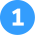How did we get here?

1. Read the problem: Using a number line, find the sum of 6 and 5.
2. Using a number line, locate the 6 and place your pencil on the line.
3. Then, make small loops along the way as you hop to the right until you count 5 places.
4. You should now be on 11 on the number line.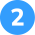How did we get here?

1. Read the problem: Fill in the blank: 12 + __ = 25
2. Using a number line, locate the 12 and place your pencil on the line.
3. Move your pencil to the right until you reach the 25, counting how many spaces are in between 12 and 25.
4. There are 13 spaces, or hops, on the number line between 12 and 25.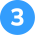How did we get here?

1. Read the problem: What is the difference between 325 and 218?
2. Rewrite the problem vertically with the larger greater on top, lining up all of the place values.
3. Use the carry the 1 method when adding the ones (the 5 and the 8) to find the sum between 5 and 8. Add that one to the tens column, making that part 1 + 2 + 1.
4. The sum of 325 and 218 is 543.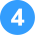How did we get here?

1. Read the problem: Julie has 5 flowers, and Kevin has 7. How many flowers do they have together?
2. Using a number line, locate the 5 and place your pencil on the line.
3. Move your pencil to the right 7 hops.
4. Your pencil will land on the 12.
5. 5 + 7 = 12.“Difference” refers to the subtraction of one number from another. It indicates the numerical distance between two values. “Sum” refers to the result of adding two or more numbers together.

To find the sum of two numbers, add the two numbers together. The result will be the combined total of the two numbers.

Regrouping, also known as carrying, is a technique used in addition when the total in one place value (like the ones or the tens) is 10 or greater. You simply take the 1 (like the 1 in 17) and add it to the next value place over to the left. This demonstrates that a number larger than 9 can be understood to be 10 + whatever is in the ones space.

To sum up, addition is a vital foundation for all math skills to follow. With a little bit of practice, you’ll soon understand this cornerstone of math and will soon be able to tackle more complex forms of arithmetic.## Related Posts

Title 1

Title 2

Title 3

Lesson credits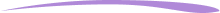Taylor Hartley

Taylor Hartley is an author and an English teacher based in Charlotte, North Carolina. When she's not writing, you can find her on the rowing machine or lost in a good novel.

Jill Padfield has 7 years of experience teaching high school mathematics, ranging from Alegra 1 to AP Calculas. She is currently working as a Business Analyst, working to improve services for Veterans while earning a masters degree in business administration.

Taylor Hartley

Taylor Hartley is an author and an English teacher based in Charlotte, North Carolina. When she's not writing, you can find her on the rowing machine or lost in a good novel.

Jill Padfield has 7 years of experience teaching high school mathematics, ranging from Alegra 1 to AP Calculas. She is currently working as a Business Analyst, working to improve services for Veterans while earning a masters degree in business administration.# Are you a parent, teacher or student?

Are you a parent or teacher?

## Hi there!

Book a chat with our team